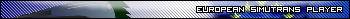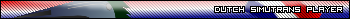## News:

Do you need help?
Simutrans Wiki Manual can help you to play and extend Simutrans. In 9 languages.

## Manhattan versus Euclidian distance

Started by jamespetts, March 20, 2009, 11:54:07 PM

0 Members and 1 Guest are viewing this topic.

#### jamespetts

When I was testing my new code for Simutrans-Experimental to calculate the average speed, I found that the average speed given was far too high (often in excess of the vehicle's maximum speed) where vehicles travelled a significant distance on the diagonal. I realised that this must be because the number of steps per tile for diagonal tiles has recently been reduced from 255 to 127, but the Manhattan distance is still used. This would give results that correlated correctly with the speed calculations when the diagonals were 255 per tile, but not with the new system where diagonals are 127 per tile. So, I wrote a new method in koord.h thus:

`tatic inline double accurate_distance(const koord &a, const koord &b){ // Euclidian distance return sqrt(pow((double)a.x - (int)b.x, 2) + pow((double)a.y - (int)b.y, 2));}`

and used that instead for my speed calculations. It gave an accurate result: the average speed of a train with a maximum speed of 300kph was 222kph, not 315kph. That made me wonder whether there are other places in the code where the Manhattan distance is being used where it would, now that the conversion to 127 step diagonal tiles has been made, be better to use the Euclidian distance. Would it be better to use it, for example, for checking the distance for the revenue calculation? I appreciate that it might be slightly slower than the Manhattan distance to calculate, but the difference is not likely to be very great, and, in any event, the method is not, I do not think, called often enough for it to make a great difference to the overall speed of the game. Are there any potential problems with replacing calls to abs_distance with calls to accurate_distance? Would it cause problems elsewhere?

Want to help with development? See here for things to do for coding, and here for information on how to make graphics/objects.

#### Combuijs

#1
QuoteI appreciate that it might be slightly slower than the Manhattan distance to calculate, but the difference is not likely to be very great

Forget about the slightly. Your version is about a 100 times slower. If you would have used (a.x -b.x)*(a.x-b.x) (or still better int xx = a.x -b.x; and then xx*xx) you might speed up things considerably, but the use of sqrt is still slowing things down a lot.

I would call the fragment (double)a.x - (int)b.x not the most beautiful statement of the year, to put it mildly.

Quotein any event, the method is not, I do not think, called often enough for it to make a great difference to the overall speed of the game

That, however, might be certainly true!
Bob Marley: No woman, no cry

Programmer: No user, no bugs#### jamespetts

#2
Combuijs,

I got the formula from several websites that I searched for finding a means of obtaining an accurate distance between two points on a grid, which required both the square root and the power operators. I do not know whether your suggested alternatives are equivalent (mathematics is not my strong point), but, of course, if there is a method that is faster and no less (or, at least, not significantly less) accurate than that above, then I'd gladly use it.

The reason that I use the casts is that the koord class uses 16-bit integers for both its values, yet there is no pow() overload that takes two integers, so I get a compiler error when not casting; there is one that takes two doubles, one that takes a double and an int, one that takes two floats, one that takes a float and an int, one that takes two long doubles and one that takes a long double and an int. If you can suggest a better way of doing it than using those casts, then I'd be very interested!

Want to help with development? See here for things to do for coding, and here for information on how to make graphics/objects.

#### ij

#3
Often sqrt could be calculated much faster than what the standard sqrt implementation does without considerable loss of accuracy. You can easily find an estimating/iterative algorithm. Alternatively, sometimes it's possible to transform the problem into x^2 space and not use sqrt at all.

#### Combuijs

#4
QuoteIf you can suggest a better way of doing it than using those casts, then I'd be very interested!

int diffx = a.x - b.x;
int diffy = a.y - b.y;
double diffx2 = diffx * diffx;
double diffy2 = diffy * diffy;
return sqrt (diffx2 + diffy2);

The doubles are needed to prevent overflow. (And that is by the way why Manhattan distance is so much easier, in no way you can get overflow and it is much more accurate). Actually, the above code can be shorter (1 line...), but this one makes clear what is happening.

Don't ever use the pow() function to square a number, it is much more inaccurate and much more slower.

QuoteAlternatively, sometimes it's possible to transform the problem into x^2 space and not use sqrt at all.

Definitely true. If for instance you want to know if the distance > 100 then you better can have a function that calculates the square euclidean distance, and then compare square_distance > 10000. Much faster, much more accurate.

Furthermore, if you use doubles you can never ever compare two distances without using a tolerance, e.g. don't use:

if (distance(a,b) == distance (c,d))

but use:

tol = 0.001; //an infinitely small number for your project
if (Abs (distance(a,b) - distance (c,d)) < tol)

or still better:

tol = 0.001; //an infinitely small number for your project
if (Abs (square_distance(a,b) - square_distance (c,d)) < tol * tol)

So, you see, by introducing doubles in your project you have to redesign lots of existing code to accomodate them.
Bob Marley: No woman, no cry

Programmer: No user, no bugs#### jamespetts

#5
Thank you both for your advice! After reading ij's post (and before reading Combuijs's latest post), I re-did the code to the following, adapting code snippets found on the web for the integer square root function:

`static inline uint32 accurate_distance(const koord &a, const koord &b){ // Euclidian distance return integer_sqrt((a.x - b.x) * (a.x - b.x)) + ((a.y - b.y) * (a.y - b.y));}static inline uint32 integer_sqrt(const uint32 num) {    if (0 == num) { // Avoid zero divide return 0; }      uint32 n = (num / 2) + 1;       // Initial estimate, never low    uint32 n1 = (n + (num / n)) / 2;    while (n1 < n) {        n = n1;        n1 = (n + (num / n)) / 2;    }    return n;}`

Is that any better? I have also removed the previously required #include<math.h> line.

Want to help with development? See here for things to do for coding, and here for information on how to make graphics/objects.

#### whoami

#6
Quote from: jamespetts on March 20, 2009, 11:54:07 PM
(...) be better to use the Euclidian distance. Would it be better to use it, for example, for checking the distance for the revenue calculation?
If another distance is going to be used, the cost calculation should be changed to use the new kind, too.

#### jamespetts

#7
Quote from: whoami on March 22, 2009, 03:52:08 PM
If another distance is going to be used, the cost calculation should be changed to use the new kind, too.

Yes, I agree. See here for details.

Want to help with development? See here for things to do for coding, and here for information on how to make graphics/objects.

#### whoami

#8
I hadn't seen that topic before posting here, but there you refer to building cost, right? Here I meant moving cost of vehicles.

#### jamespetts

#9
Ahh, good point... how would that be changed, do you think?

Want to help with development? See here for things to do for coding, and here for information on how to make graphics/objects.

#### whoami

#10
Vehicle movement knows about travelling over a diagonal, so it should inform the cost counter to deduct only the appropriate fraction. Calculating this for every tile is not useful, so the differing value should be calculated beforehand, and kept in a different variable (per convoy, I guess).

#### jamespetts

#11
Good idea! I'll look into that. (Incidentally - where in the code is the part that detects diagonals?)

Edit: I have found what I am looking for, and the resulting code is here:

`voidvehikel_t::hop(){ // Fahrtkosten // "Travel costs" (Babelfish) uint16 costs = besch->get_betriebskosten(welt); if(costs != base_costs) { // Recalculate base costs only if necessary // With this formula, no need to initialise base // costs or diagonal costs! base_costs = costs; diagonal_costs = (costs * diagonal_length) / 255; } if(steps_next != 255) { costs = diagonal_costs; } cnv->add_running_cost(-costs); ...`

Now to find a way of doing that with the purchase and maintenance cost for the ways... any tips, anyone?